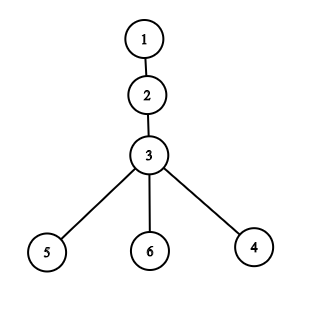# Signed integer overflow for no apparent reason

Hi,
I submitted this solution1 and this solution2 for this plroblem.
As you can see they received a WA and diagnostic hint that there might be an integer overflow.
But the 32-bit signed int holds values well above the given constraints of n (ie.2* 10^5) then why is there an int overflow.
Thanks

the values held by the tree is 2* 10^5 as well.
So if all elements have the value as 2 * 10^5 the final integer would be larger than INT causing overflow.
I suggest changing it to long or long long will be sufficient enough to hold the answer as the max value cannot exceed 4 * 10^10 which is well under the limit of long long or long.

2 Likes

The answer for this graph and k=3Is 9. Now imagine, instead of 3 leaf nodes, there were 10^5 leaf nodes, and k=10^5 The answer would be 10^{10}, which would overflow an int

4 Likes

Thanks now I understand